Pay with
Markets
Derivatives
Earn
NFT
Institutional
Feed

# FAQAccount FunctionsTutorialBinance Fan TokenBinance EarnCrypto Deposit/Withdrawal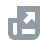Crypto Derivatives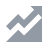FinanceAPISecurityOther Topics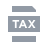Tax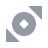Binance Convert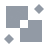NFTVIP
Home
Support Center
FAQ
How to Calculate Margin Liquidation Price

# How to Calculate Margin Liquidation Price

2021-04-08 01:40
The formula for calculating the liquidation price for a margin trade using currency "i" is as follows:
• "i" represents the currency "i";
• "Ai" represents the total amount of "i" assets;
• "Li" represents the borrowed amount of "x" asset;
• "Ri" represents the amount of interest payable on "x" asset;
• "Pi" represents the index price of the "x" asset/BTC (or USDT) pair;
• "li" represents the liquidation price for "x" asset.
Liquidation will be triggered when the risk ratio reaches the liquidation risk ratio.
Risk Ratio = Total Assets / (Total Amount Borrowed + Interest Payable).
Using the "i" currency as an example:
Therefore, the liquidation reference price for "i" currency is:
Ratio of the Index Price to the Liquidation Reference Price = (Liquidation Price - Index Price) / Index Price.